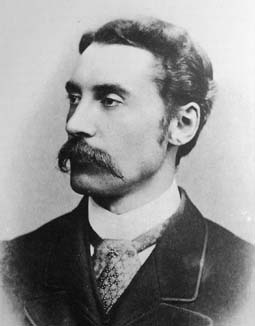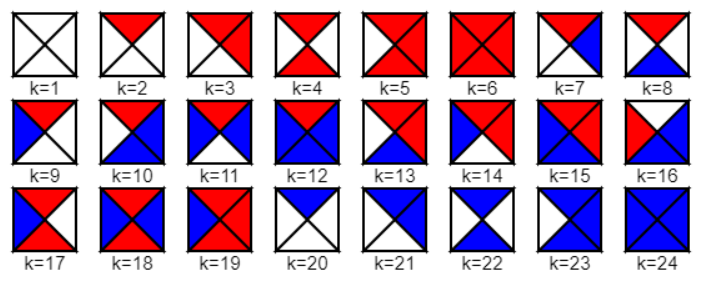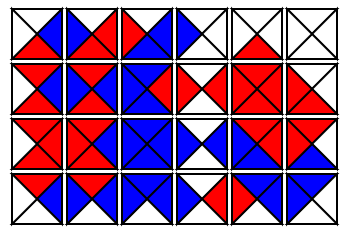## Sunday, December 26, 2021

### MacMahon SquaresPercy Alexander MacMahon 

This post is about a puzzle designed by Percy Alexander MacMahon  and described in a book published in 1921 .

We consider a collection of 24 squares, where the four edges (top, right, bottom, left) are colored by three different colors.MacMahon Squares

These 24 squares are all the squares we can make this way using three colors. Note that rotation does not give us a new square. The number of squares we can create using $$n$$ colors is : $\frac{n(n+1)(n^2-n+2)}{4}$ This formula is not obvious to me.

These 24 squares need to be placed on a $$4 \times 6$$ grid (allowing rotation), subject to the following constraints:

• The edges at the border of the grid should be white
• The touching edges of two neighboring squares should have the same color

The best is to show a feasible solution:A single feasible solution

My first task: try to develop a mathematical programming model that finds a single feasible solution. For this we use a bunch of sets:

• $$i \in \{1,\dots,4\}$$ indicates the row in the grid,
• $$j \in \{1,\dots,6\}$$ is the column,
• $$k \in \{1,\dots,24\}$$ is the square,
• $$r \in \{0^{\circ},45^{\circ},90^{\circ},135^{\circ}\}$$ is the rotation applied,
• $$c \in \{\color{darkgreen}{\mathit{White}},\color{darkgreen}{\mathit{Red}},\color{darkgreen}{\mathit{Blue}}\}$$ are the colors,
• $$e \in \{\color{darkgreen}{\mathit{Top}},\color{darkgreen}{\mathit{Right}},\color{darkgreen}{\mathit{Bottom}},\color{darkgreen}{\mathit{Left}}\}$$ is an edge,
• $$\color{darkblue}{\mathit{Sq}}_{k,r,e,c}$$ is the color for an edge after rotation of square $$k$$. This describes our collection of squares,
• $$\color{darkblue}{\mathit{Border}}_{i,j,e}$$ identifies the edges that form the outer border of the grid,
• $$\color{darkblue}{\mathit{Touch}}_{i,j,e,i',j',e'}$$ gives us the edges of neighboring cells in the grid that touch each other.

I introduce two variables:  $\color{darkred}x_{i,j,k,r} = \begin{cases} 1 & \text{if square k is placed at (i,j) after rotation}\\ 0 & \text{otherwise}\end{cases}$ and $\color{darkred}y_{i,j,e,c} = \begin{cases} 1 & \text{if edge e for the square at (i,j) has color c}\\ 0 & \text{otherwise}\end{cases}$

We have fairly standard assignment constraints: \begin{align} & \sum_{i,j,r} \color{darkred}x_{i,j,k,r} = 1 && \forall k \\ & \sum_{k,r} \color{darkred} x_{i,j,k,r} = 1 && \forall i,j \end{align} The variables $$\color{darkred}x$$ and $$\color{darkred}y$$ are linked as follows: $\color{darkred}y_{i,j,e,c} = \sum_{k,r|\color{darkblue}{\mathit{Sq}}(k,r,e,c)} \color{darkred}x_{i,j,k,r} \>\> \forall i,j,e,c$ The constraint to fix the color of the edges at the border to White is: $\color{darkred}y_{i,j,e,\color{darkgreen}{\mathit{White}}}= 1 \>\> \forall \color{darkblue}{\mathit{Border}}_{i,j,e}$ Finally the constraint to align colors where edges of neighboring squares touch each other: $\color{darkred}y_{i,j,e,c} = \color{darkred}y_{i',j',e',c} \>\>\forall \color{darkblue}{\mathit{Touch}}_{i,j,e,i',j',e'}$

MIP Model
\begin{align}& \sum_{i,j,r} \color{darkred}x_{i,j,k,r} = 1 && \forall k \\ & \sum_{k,r} \color{darkred} x_{i,j,k,r} = 1 && \forall i,j \\ &\color{darkred}y_{i,j,e,c} = \sum_{k,r|\color{darkblue}{\mathit{Sq}}(k,r,e,c)} \color{darkred}x_{i,j,k,r} && \forall i,j,e,c \\ &\color{darkred}y_{i,j,e,\color{darkgreen}{\mathit{White}}}= 1 && \forall \color{darkblue}{\mathit{Border}}_{i,j,e} \\ & \color{darkred}y_{i,j,e,c} = \color{darkred}y_{i',j',e',c} && \forall \color{darkblue}{\mathit{Touch}}_{i,j,e,i',j',e'}\\ & \color{darkred}x_{i,j,k,r} \in \{0,1\} \\ & \color{darkred}y_{i,j,e,c} \in \{0,1\} \end{align}

Notes:

• There is no objective: we only want to find feasible solutions.
• The $$\color{darkred}y$$ variable can be declared as continuous between 0 and 1. It will be integer automatically.
• We can reduce the size of the model a bit by being more clever about the set $$\color{darkblue}{\mathit{Sq}}_{k,r,e,c}$$. For squares with just one color, there can be just one rotation: the other ones don't add anything.  For some squares with 2 colors, like square $$k=4$$, there are just two meaningful rotations. The number of elements for $$\color{darkblue}{\mathit{Sq}}$$ will decrease from 384 to 324.

#### All solutions

There are many solutions to this problem. We can find some of them by adding "no-good cuts" to the problem that forbids an earlier found solution (but nothing else). For this we store previous solutions in a parameter $\color{darkblue}x^*_{s,i,j,k,r} \in \{0,1\}$ where $$s$$ is the solution number. Each solution has exactly $$\textbf{card}(k)=24$$ ones. Our cut can look like: $\sum_{i,j,k,r} \color{darkblue}x^*_{s,i,j,k,r} \cdot \color{darkred}x_{,i,j,k,r} \le \textbf{card}(k)-1\>\>\forall s$ Here is a picture of ten solutions found this way:

Note. We can see that square number $$k=1$$ (all white edges) is always at the border. This can be proven as follows :
1. There are 16 border positions and there are 18 squares with at least one white edge. So exactly two interior cells will contain a square with a white cell.
2. Square $$k=1$$ in the middle requires two or three interior neighbors with white edges. From 1 we know we only have one such square available.

The total number of solutions is quite large. In 1964, a computer program was used to enumerate all solutions . It was written in Algol, and ran on a B5000.  Their answer was: 12,261 (excluding symmetries).

As the number of solutions is so large, it makes sense to look into using the solution pool. When I use the solution pool, Cplex reports 106,624 solutions. This includes symmetric versions. I am not sure how we can bridge the gap from 12,261 to 106,624. I expected some multiple of 12,261 but that is not the case here.

#### Lingering questions

Some questions remain:

• How to derive the formula for the number of squares for $$n$$ colors?
• How can we reproduce the total number of solutions?
• Can we generate the collection of MacMahon squares automatically, that is using code or by a model?

#### References

1. Percy Alexander MacMahon, https://en.wikipedia.org/wiki/Percy_Alexander_MacMahon
2. MacMahon, Percy (1921). New Mathematical Pastimes. Cambridge University Press.
3. MacMahon Squares, https://en.wikipedia.org/wiki/MacMahon_Squares
4. Feldman, Gary, Documentation of the MacMahon Squares Problem. AIM-012, Stanford Artificial Intelligence Laboratory, 1964, https://exhibits.stanford.edu/stanford-pubs/catalog/nv052jg0055

#### Appendix: GAMS code

 $ontext https://en.wikipedia.org/wiki/MacMahon_Squares We have 24 different squares with 4 colored edges, as in: _ _ |\ W /| |R\ / | | /W\B | |/_ _\ | Place these 24 squares on a 4 x 6 grid. Squares can be rotated. Constraints: border of grid has white edges touching edges have the same color Q: how many ways to place squares on the grid.$offtext $set htmlfile MacMahon.html$set jsfile   data.js *-------------------------------------------------------------- * data *-------------------------------------------------------------- sets    i 'rows'      /row1*row4/    j 'columns'   /col1*col6/    k 'square'    /square1*square24/    r 'rotation'  /0deg,45deg,90deg,135deg/    c 'colors'    /Red,White,Blue/    e 'edge'      /top,right,bottom,left/    sq(k,r,e,c) 'squares' ; acronym Wh,Rd,Bl; parameter    Colors(c) /Red Rd, White Wh, Blue Bl/    Rotate(r) 'how many 45 degree rotations' ; Rotate(r) = ord(r)-1; table Squares(k,e)           top  right bottom left square1    Wh    Wh    Wh    Wh square2    Rd    Wh    Wh    Wh square3    Rd    Rd    Wh    Wh square4    Rd    Wh    Rd    Wh square5    Rd    Rd    Rd    Wh square6    Rd    Rd    Rd    Rd square7    Rd    Bl    Wh    Wh square8    Rd    Wh    Bl    Wh square9    Rd    Wh    Wh    Bl square10   Rd    Bl    Bl    Wh square11   Rd    Bl    Wh    Bl square12   Rd    Bl    Bl    Bl square13   Rd    Rd    Bl    Wh square14   Rd    Rd    Wh    Bl square15   Rd    Rd    Bl    Bl square16   Wh    Bl    Bl    Rd square17   Rd    Wh    Rd    Bl square18   Rd    Bl    Rd    Bl square19   Rd    Rd    Rd    Bl square20   Bl    Wh    Wh    Wh square21   Bl    Bl    Wh    Wh square22   Bl    Wh    Bl    Wh square23   Bl    Bl    Bl    Wh square24   Bl    Bl    Bl    Bl ; sq(k,r,e,c) = Squares(k,e--rotate(r))=colors(c); option sq:0:0:4; display sq; parameter numsq(*) 'number of elements in sq (w or w/o duplicates)'; numsq('with dups') = card(sq); display "with duplicates",sq; *-------------------------------------------------------------- * remove duplicates from rotations * this is not so important for finding a single solution * but it is for enumerating all solutions. *-------------------------------------------------------------- * flag, set to 0 or 1 scalar RemoveDuplicates /1/; alias(r,rr); scalar diff; loop(k$RemoveDuplicates, loop(r$(ord(r)>1),      loop(rr$(ord(rr)1), solve m2 minimizing z using mip; xsol(sol,i,j,kr) = round(x.l(i,j,kr)); ysol(sol,i,j,e,c) = round(y.l(i,j,e,c)); s(sol) = yes; ); display xsol; *-------------------------------------------------------------- * Generate picture of solutions in HTML/JS *-------------------------------------------------------------- file f /'%jsfile%'/; put f; * collection of squares put "Squares=["/; loop(k, put " ["; loop(sq(k,'0deg',e,c),put '"',c.tl:0,'",'); put "],"/; ); put "];"/; * solutions put "Solutions=["/; loop(sol, put "["; loop((i,j,kr)$xsol(sol,i,j,kr),      put ' {row:',ord(i):0:0,',col:',ord(j):0:0;      put ',square:[';      loop((e,c)$ysol(sol,i,j,e,c), put '"',c.tl:0,'",'; ); put "]},"/; ); put "],"/; ); putclose "];"; *$libInclude win32 shellexecute  "%htmlfile%" * for older gams systems use: execute 'shellexecute "%htmlfile%"'; *-------------------------------------------------------------- * Use solution pool to enumerate all solutions *-------------------------------------------------------------- option threads=8, mip=cplex; m.optfile=1; Solve m using mip minimizing z ; $onecho > %htmlfile% MacMahon Squares MacMahon Squares Collection of squares Subset of solutions$offecho $onecho > cplex.opt SolnPoolIntensity = 4 PopulateLim = 200000 SolnPoolPop = 2 solnpoolmerge solutions.gdx$offecho

Notes:
• Acronyms are used to make the input table more compact.
• The \$onecho constructs are placed at the bottom to keep them out of the way. Notice however that this is a compile-time comment. So even this is at the end of the file, it will be performed before executing any other statements.

#### 1 comment:

1.The formula for the number of n-colorings up to rotation arises from Burnside's Lemma. The identity element (rotation by 0 degrees) fixes n^4 colorings, rotation by 90 degrees or 270 degrees fixes n colorings each, and rotation by 180 degrees fixes n^2 colorings, yielding (n^4+2n+n^2)/4.